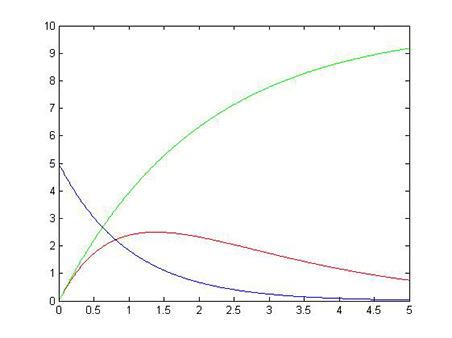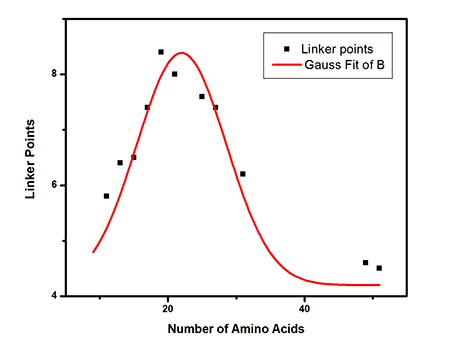# Team:UESTC Life/Modeling

Modeling

## First Model

Our first model explored the change of intermediate product in multistep degradation of TCP. assume K1=1，K2=0.5,the reaction is TCP→2,3DCP+Cl^- ; 2,3DCP→A+Cl^-. Through matlab analyzing, the program was in the program bar. Finally, the reaction curve was predicted. The result was similar to what we had got through experiment.FIG. The blue line stands for TCP; Red line stands for 2,3-DCP; Green line stands for serial product after 2,3-DCP.

## Second Model

The second model grade Linker and predict optimal length of linker in chimeric protein. According to many papers, the effects of linker length on equilibrium stability arise from significant and sometimes opposing changes in folding and unfolding kinetics.[1,4,7,8] As for the structure, the rank of linker is α-helix >β-sheet > loop.[2,3,4,5,8] And the effect of structure is dominant. We collected amass of data1,[4,6,7,8] and degraded the effect of linker. Finally, we got a formula. The formula can predict the efficiency of the length of linker. The full mark is 10 points.

### Length pointsy=y0 + (A/(w*sqrt(PI/2)))*exp(-2*((x-xc)/w)^2)

Y0=4.19829; Xc=21.97825; W=13.10889; A=68.84675

### Structure point

1.α-helix A 2.β-sheet B 3. loop C

### Results

The point of P2A is A, the length point is 7.9777
P2A is in high score in this system. It is similar to our experiment result.

### Predict

The optimal linker is an α-helix and the number of amino acid is 22. So in future work we can construct new chimeric protein using this linker.